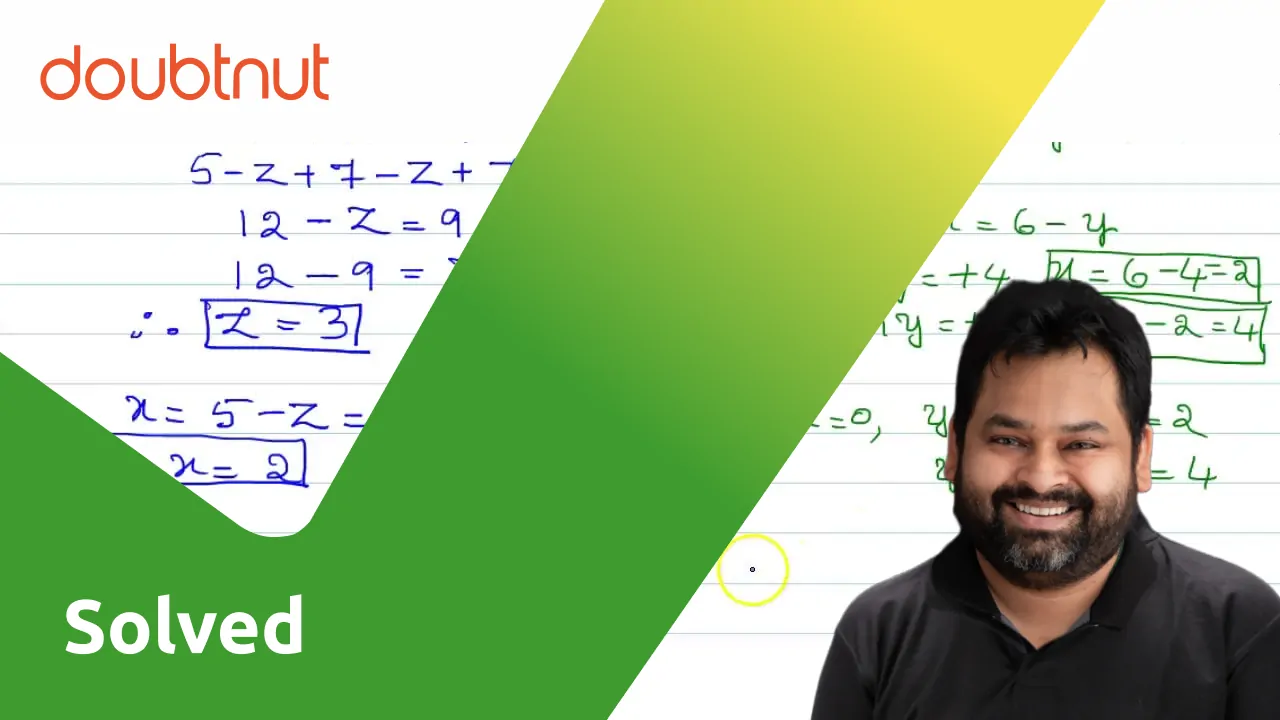Doubtnut App - Get 50 Lakh+ video solutions better than GoogleInstall Now

# Find the value of x, y and z from the following equations: (i) [43x5]=[yz15] (ii) [x+y25+zxy]= (iii) ⎡⎢⎣x+y+zx+zy+z⎤⎥⎦=⎡⎢⎣957⎤⎥⎦ Solution in Kannada

Text Solution
Verified by Experts

## The correct Answer is:(i) x=1,y=4,z=3 (ii) x=4,y=2,z=0orx=2,y=4,z=0 (iii) x=2,y=4,z=3

Video Solution